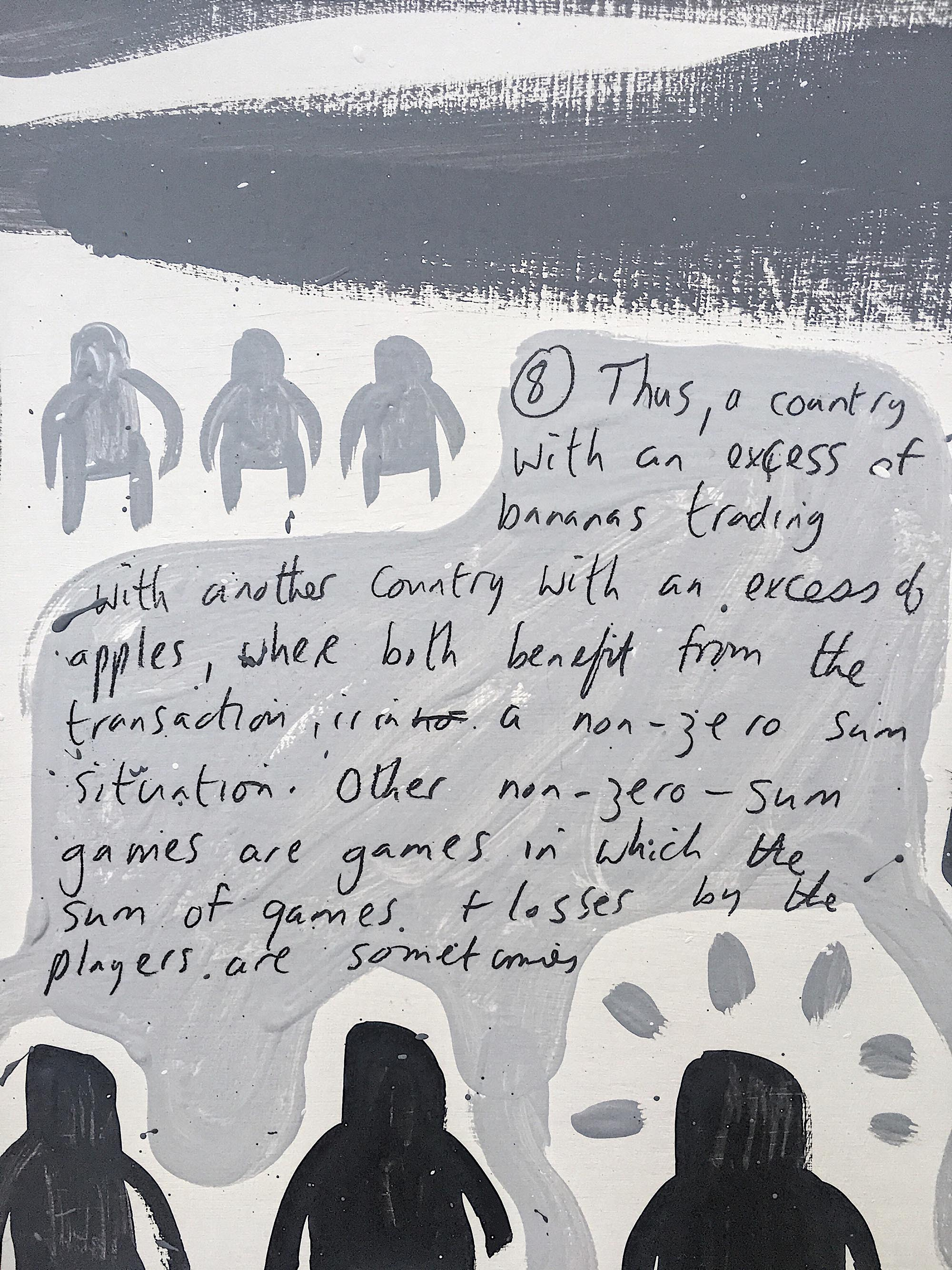#  Zero Sum Game

Completion Date: 22 August 2019
Medium: Paint on card
Dimensions: 16 x 24 inches

In game theory and economic theory, a zero-sum game is a mathematical representation of a situation in which each participant’s gain or loss of utility is exactly balanced by the losses or gains of the utility of the other participants. If the total gains of the participants are added up and the total losses are subtracted, they will sum to zero. Thus, when cutting a pizza, where taking a larger piece reduces the amount of pizza available for others, is a zero-sum game if all participants value each unit of pizza equally.